# linear equations in two variables class 9 worksheet

Given below are the Linear equations in two variables class 9 worksheet with solutions
(a) Concepts questions
(b) Calculation problems
(c) Multiple choice questions
(e) Fill in the blank's

Question 1
Draw the graph of each of the equations given below. Also, find the co-ordinates of the point where the graph cuts the co-ordinates axis:
(a) 6x - 4y = 12
(b) 4y -x= 8
Solution
To draw the graph, we need at least two solutions of the equation We can get two points by putting x=0 and y=0. And then draw the graph. Corrdinates will be given by (x,0) and (0,y)

a.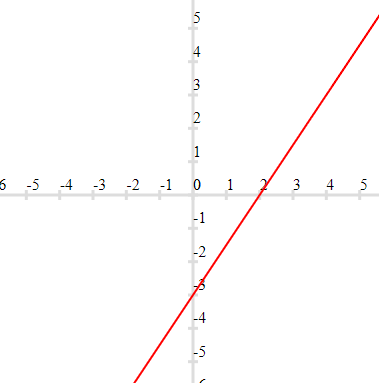Coordinates are (2,0) and (0,-4)

b.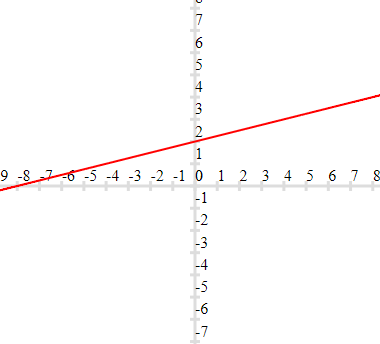Coordinates are (-8,0) and (0,2)

Question 2
The taxi fare in a delhi is as follows: For the first kilometer, the fare is Rs16 and for the subsequent distance it is Rs 10/km. Taking the distance covered as x km and total fare as Rs y, write a linear equation for this information, and draw its graph.
Solution
Total fare = Fare of first kilometer + 10 × subsequent distance travelled
$y= 16 + 10 (x-1)$
$y=16 + 10x- 10$
$y=6+10x$
We can draw the graph by putting points x=0 and x=1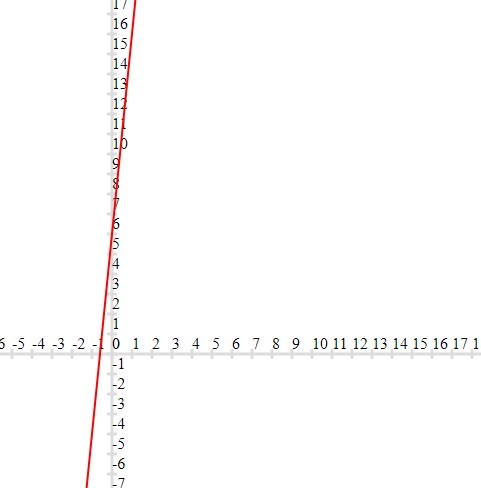Question 3
If a linear equation has solutions (-2, 2), (0, 0) and (2, -2), then it is of the form
(a) y - x = 0
(b) x + y = 0
(c) -2x + y = 0
(d) -x + 2y = 0
Solution

Question 4
Which of the below statement is true
(a) A linear equation x + 3y = 5 has a unique solution
(b) The graph of the equation y = x + 1 passes through the origin
(c) The line parallel to the y-axis at a distance 4 units to the left of y-axis is given by the equation y = - 4
(d) None of the above
Solution

Question 5
Any point on the y-axis is of the form
(a) (x, 0)
(b) (x, y)
(c) (0, y)
(d) ( y, y)
Solution

Question 6
Draw the graph of the equation 2x + 3y = 12. From the graph, find the coordinates of the point:
Whose y- coordinate is 3
Whose x- coordinate is -3.

Question 7
Draw the graph of the equation 2x + y = 6. Shade the region bounded by the graph and the co- ordinate axis. Also find the area of shaded region.
Solution
The graph of the equation 2x + y = 6 can be drawn by choosing points x=0 i.e y=6 and y=0 i.e x=3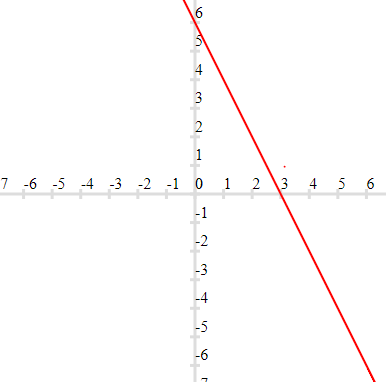Shading the region bounded by the graph and the co- ordinate axis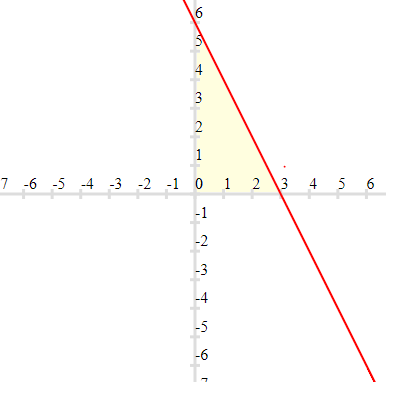This is a right angle triangle with base =3 and height =6
So area = $\frac {1}{2} \times 6 \times 3 = 9$units

Question 8
Draw the graph of the equation $\frac {x}{2} + \frac {y}{3} =1$. Also find the area of triangle formed by the line and the co-ordinates axis.
Solution
The graph of the Linear equation $\frac {x}{2} + \frac {y}{3} =1$ can be drawn by choosing points x=0 i.e y=3 and y=0 i.e x=2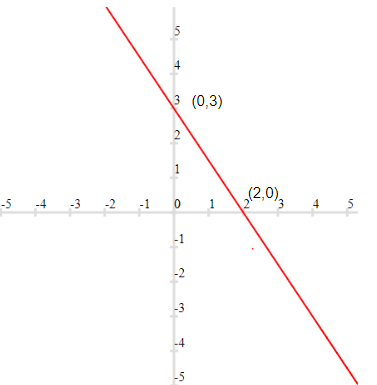Now the triangle formed by the line and co-ordinates axis is given as below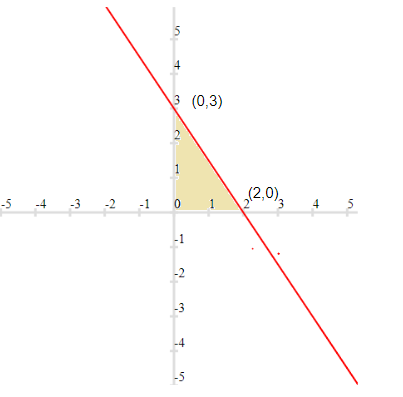This is a right angle with base as 2 units and height ad 3 units.
So ,Area = $\frac {1}{2} \times 2 \times 3= 3$ units

Question 9
Draw the graph of y = x + 2
Solution
We can get two points by putting x=0 and y=0. And then draw the graph. Corrdinates will be given by (x,0) and (0,y)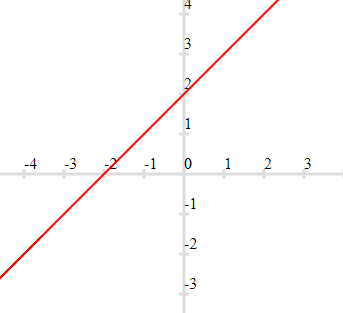Question 10
Draw the graphs of the linear equations 4x - 3y + 4 = 0 and 4x + 3y - 20 = 0. Find the area bounded by these lines and x = axis.
Solution
The graph of 4x - 3y + 4 = 0 by putting y=0 i.e x= -1 and x=2 i.e y =4
Similarly the graph 4x + 3y - 20 = 0 by putting y=0 i.e x=5 and x=2 i.e y=4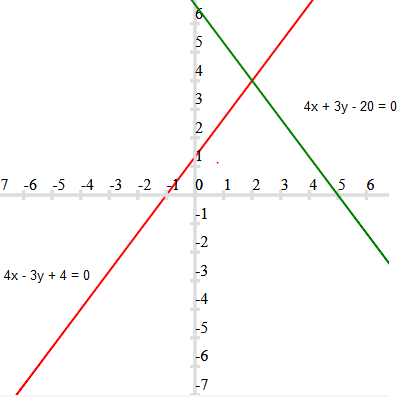Now we can see that area bounded by these lines and x = axis is a triangle with base = 6 unit and height =4 units.
So area = $\frac {1}{2} \times 6 \times 4 = 12$units

Question 11
Solve for y:
$\frac {3y+2}{7} + \frac {4 (y+1)}{5}= \frac {2}{3} (2y+1)$

Question 12
The angles of a triangle are 5x, 3 (2x - 5) and 9x -5 Find x and also name the triangle.
Solution
We know that sum of the angles in the triangle is equal to 180
Therefore,
$5x + 3(2x - 5) + 9x -5=180$
$20 x -20 =180$
$x= 10$
Therefore angles are
50°, 45° , 85°
The name of the triangle is scalene triangle

Question 13
Determine the point on graph of equation 2x + 5y = 20 whose x - co-ordinate is 5/2 times its ordinate.

Question 14
If (2a,a-1) is a solution of 2x + 3y - 9 = 0, find a
Solution
If (2a,a - 1) is the solution ,then putting x=2a and y=a-1
$2(2a) + 3(a-1) -9=0$
$4a + 3a -3 -9=0$
$a= 12/7$

Question 15
Draw the graph of linear equation x = 3y
Solution
We can draw the graph by joining for points (0,0) and (3,1)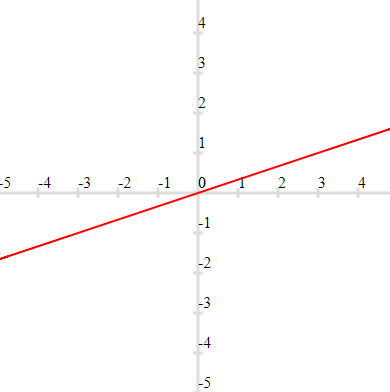Question 16
Draw the graph of linear equation 2x -9 = 0
Solution
This can be treated as equation in two variable as $2x + 0 \times y -9=0$
Drawing the graph by putting y=0 and y=2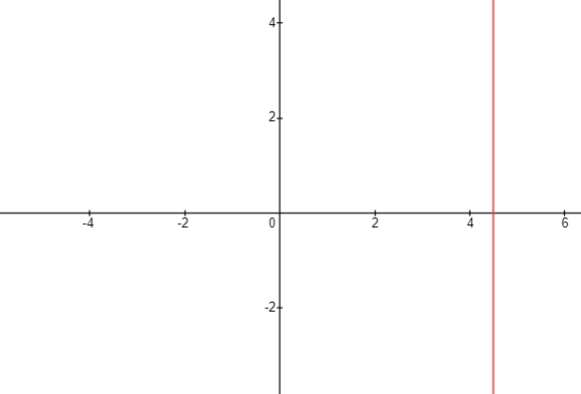Question 17
Draw the graph of linear equation x = y
Solution
We can draw the graph by joining for points (0,0) and (1,1)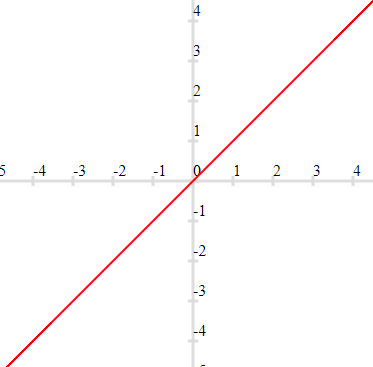Question 18
Draw the graphs of linear equation y = x and y = -x on the same Cartesian plane, what do you observe?
Solution
Here is the graph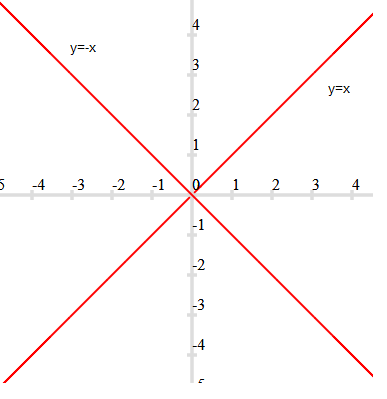We can observe that both the graph are symmetrical on both the x-axis and y-axis

Question 19
Find three different solutions of the equation 4x + 3y = 12 from its graph?

Question 20
Draw the graph of the equation x + 2y = 9. Find the vertices of the triangle formed by the line and the co-ordinate axis. Find two more points other than the intercepts on the axis.

Question 21
Draw the graph of the line x - 2y = 3. From the graph, find the co-ordinate of the point when
x = 5
y = -2

## Summary

This Linear equations in two variables class 9 worksheet with solutions is prepared keeping in mind the latest syllabus of CBSE . This has been designed in a way to improve the academic performance of the students. If you find mistakes , please do provide the feedback on the mail. You can download this test as pdf also as belowGo back to Class 10 Main Page using below links

### Practice Question

Question 1 What is $1 - \sqrt {3}$ ?
A) Non terminating repeating
B) Non terminating non repeating
C) Terminating
D) None of the above
Question 2 The volume of the largest right circular cone that can be cut out from a cube of edge 4.2 cm is?
A) 19.4 cm3
B) 12 cm3
C) 78.6 cm3
D) 58.2 cm3
Question 3 The sum of the first three terms of an AP is 33. If the product of the first and the third term exceeds the second term by 29, the AP is ?
A) 2 ,21,11
B) 1,10,19
C) -1 ,8,17
D) 2 ,11,20